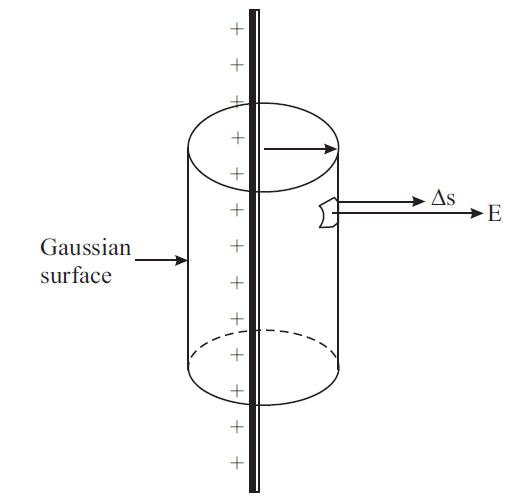# Electric Field due to a Long Line Charge – formula derivation

Last updated on May 13th, 2022 at 05:10 am

A line charge is in the form of a thin charged wire of infinite length with a uniform linear charge density σ (charge per unit length). Let there be a charge +q on the wire.

We have to calculate the electric field at a point P at a distance r.

Draw a right circular cylinder of radius r with the long wire as the axis of the cylinder.

The cylinder is closed at both ends. The surface of this cylinder is the gaussian surface and is shown in Fig. 1. The magnitude of the electric field E is the same at every point on the curved surface of the cylinder because all points are at the same distance from the charged wire.

The electric field direction and the normal to area element Δs are parallel.Fig. 1: Electric field due to an infinite line of charges having uniform linear charge density. The gaussian surface is a right circular cylinder.

Let the length of the Gaussian cylinder be l. The total charge enclosed in the cylinder is q = σ l. The area of the curved surface of the cylinder is 2 πrl.
For the flat surfaces at the top and bottom of the cylinder, the normals to these areas are perpendicular to the electric field (cos 90° = 0). These surfaces, therefore, do not contribute to the total flux. Hence

φE = Σ E. Δs
= E × 2 πrl

According to Gauss’ law, φE = q/ε0. Hence

E × 2 πrl = q/ε0

=> E × 2 πrl = ƛ l/ε0 [ Here, q = ƛl where ƛ = charge per unit length]

or E = ƛ l/(ε0 2 πrl ) = ƛ /(2 πε0r )

E = ƛ /(2 πε0r ) where ƛ = charge per unit length, and r = distance of the test point from the line of charge
(Electric Field due to a Long Line Charge at a distance r : formula derivation)

See also  Numerical problems based on the motion of a charge in a uniform electric field between two parallel plates

This shows that the electric field varies inversely with distance. (figure 2)